The first approach to generate a virtual species consists in defining its response functions to each of the environmental variables contained in our RasterStack. These responses are then combined to calculcate the environmental suitability of the virtual species.

The function providing this approach is generateSpFromFun.

## 2.1. An introduction example

Before we start using the package, let’s prepare our first simulation of virtual species.

We want to generate a virtual species with two environmental variables, the annual mean temperature bio1 and annual mean precipitation bio2. We want to generate a tropical species, i.e., living in hot and humid environments. We can define bell-shaped response functions to these two variables, as in the following figure:

## Loading required package: raster
## Loading required package: sp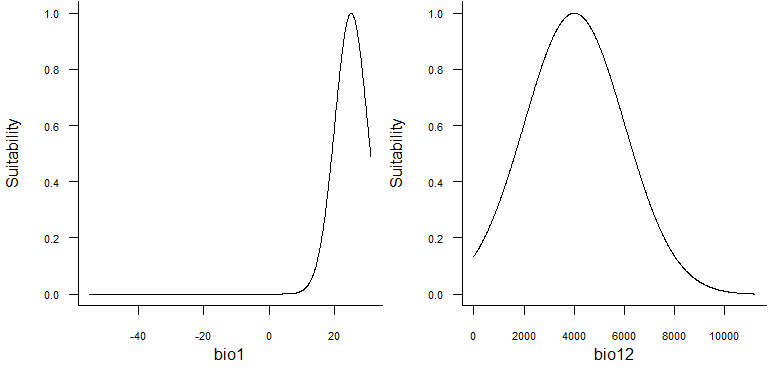Fig. 2.1 Example of bell-shaped response functions to bio1 and bio2, suitable for a tropical species.

Note that bioclimatic temperature variables (here bio1) are is in °C * 10, as explained here.

These bell-shaped functions are in fact gaussian distributions functions of the form:

$f(x, \mu, \sigma) = \frac{1}{\sigma\sqrt{2\pi}}\, e^{-\frac{(x - \mu)^2}{2 \sigma^2}}$

If we take the example of bio1 above, the mean $$\mu$$ is equal to 250 (i.e., 25°C) and standard deviation $$\sigma$$ is equal to 50 (5°C). Hence the response function for bio1 is:

$f(bio1) = \frac{1}{50\sqrt{2\pi}}\, e^{-\frac{(bio1 - 250)^2}{2 \times 50^2}}$

In R, it is very simple to obtain the result of the equation above, with the function dnorm:

# Suitability of the environment for bio1 = 15 °C
dnorm(x = 150, mean = 250, sd = 50)
##  0.001079819

The idea with virtualspecies is to keep the same simplicity when generating a species: we will use the dnorm function to generate our virtual species.

Let’s start with the package now: The first step is to provide to the helper function formatFunctions which responses you want for which variables:

library(virtualspecies)

my.parameters <- formatFunctions(bio1 = c(fun = 'dnorm', mean = 250, sd = 50),
bio12 = c(fun = 'dnorm', mean = 4000, sd = 2000))

After that, the generation of the virtual species is fairly easy:

# Generation of the virtual species
my.first.species <- generateSpFromFun(raster.stack = worldclim[[c("bio1", "bio12")]],
parameters = my.parameters,
plot = TRUE)
## Generating virtual species environmental suitability...
##  - The response to each variable was rescaled between 0 and 1. To
##             disable, set argument rescale.each.response = FALSE
##  - The final environmental suitability was rescaled between 0 and 1.
##             To disable, set argument rescale = FALSEFig. 2.2 Environmental suitability of the generated virtual species

my.first.species
## Virtual species generated from 2 variables:
##  bio1, bio12
##
## - Approach used: Responses to each variable
## - Response functions:
##    .bio1  [min=-269; max=314] : dnorm   (mean=250; sd=50)
##    .bio12  [min=0; max=9916] : dnorm   (mean=4000; sd=2000)
## - Each response function was rescaled between 0 and 1
## - Environmental suitability formula = bio1 * bio12
## - Environmental suitability was rescaled between 0 and 1

Congratulations! You have just generated your first virtual species distribution, with the default settings. In the next section you will learn about the simple, yet important settings of this function.

## 2.2. Customisation of parameters

The function generateSpFromFun proceeds into four important steps:

1. The response to each environmental variable is calculated according to the user-chosen functions.
2. Each response is rescaled between 0 and 1. This step can be disabled.
3. The responses are combined together to compute the environmental suitability. The user can choose how to combine the responses.
4. The environmental suitability is rescaled between 0 and 1. This step can be disabled.

### 2.2.1. Response functions

You can use any existing function with generateSpFromFun, as long as you provide the necessary parameters. For example, you can use the function dnorm shown above, if you provide the parameters mean and sd: formatFunctions(bio1 = c(fun = 'dnorm', mean = 250, sd = 50)). Naturally you can change the values of mean and sd to your needs.

You can also use the basic functions provided with the package:

• Linear function: formatFunctions(bio1 = c(fun = 'linearFun', a = 1, b = 0)) $f(bio1) = a * bio1 + b$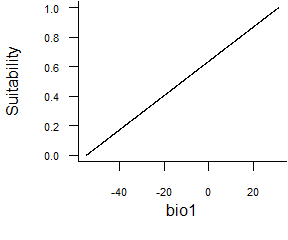Fig. 2.3 Linear response function

• Quadratic function: formatFunctions(bio1 = c(fun = 'quadraticFun', a = -1, b = 2, c = 0)) $f(bio1) = a \times bio1^2 + b \times bio1 + c$• Logistic function: formatFunctions(bio1 = c(fun = 'logisticFun', beta = 150, alpha = -5)) $f(bio1) = \frac{1}{1 + e^{\frac{bio1 - \beta}{\alpha}}}$Fig. 2.5 Logistic response function

• Normal function defined by extremes: formatFunctions(bio1 = c(fun = 'custnorm', mean = 250, diff = 50, prob = 0.99)) This function allows you to set extremum of a normal curve. In the example above, we define a response where the optimum is 25°C (mean = 250), and 99% of the area under the curve (prob = 0.99) will be comprised between 20 and 30°C (diff = 50).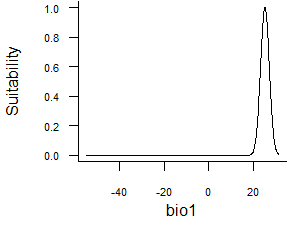Fig. 2.6 Normal function defined by extremes

• Beta response function (Oksanen & Minchin, 2002, Ecological Modelling 157:119-129): formatFunctions(bio1 = c(fun = 'betaFun', p1 = 0, p2 = 250, alpha = 0.9, gamma = 0.08)) $f(bio1) = (bio1 - p1)^{\alpha} * (p2 - bio1)^{\gamma}$Fig. 2.7 Beta response function

• Or you can create your own functions (see the section How to create your own response functions if you need help for this).

### 2.2.2. Rescaling of individual response functions

This rescaling is performed because if you use very different response function for different variables, (e.g., a Gaussian distribution function with a linear function), then the responses may be disproportionate among variables. By default this rescaling is enabled (rescale.each.response = TRUE), but it can be disabled (rescale.each.response = FALSE).

### 2.2.3. Combining response functions

There are three main possibilities to combine response functions to calculate the environmental suitability, as defined by the parameters species.type and formula:

• species.type = "additive": the response functions are added.
• species.type = "multiplicative": the response functions are multiplied. This is the default behaviour of the function.
• formula = "bio1 + 2 * bio2 + bio3": if you choose a formula, then the response functions are combined according to your formula (parameter species.type is then ignored).

For example, if you want to generate a species with the same partial responses as in 2.1, but with a strong importance for temperature, then you can specify the formula : formula = "2 * bio1 + bio12"

library(virtualspecies)

my.parameters <- formatFunctions(bio1 = c(fun = 'dnorm', mean = 250, sd = 50),
bio12 = c(fun = 'dnorm', mean = 4000, sd = 2000))

# Generation of the virtual species
new.species <- generateSpFromFun(raster.stack = worldclim[[c("bio1", "bio12")]],
parameters = my.parameters,
formula = "2 * bio1 + bio12",
plot = TRUE)
## Generating virtual species environmental suitability...
##  - The response to each variable was rescaled between 0 and 1. To
##             disable, set argument rescale.each.response = FALSE
##  - The final environmental suitability was rescaled between 0 and 1.
##             To disable, set argument rescale = FALSE
##  "2 * bio1 + bio12"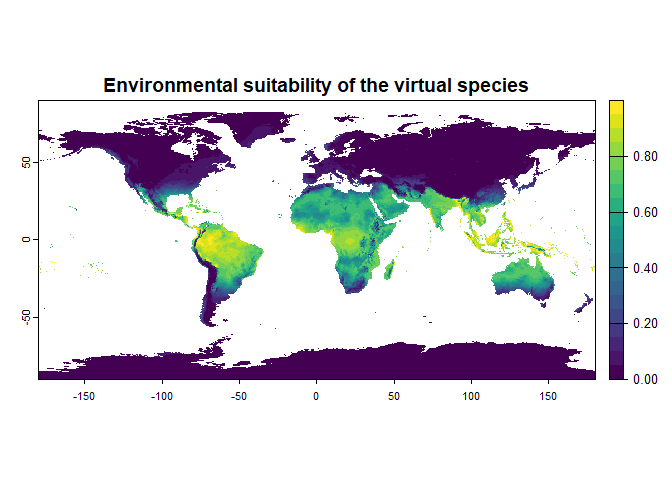Fig. 2.3 Environmental suitability of the generated virtual species

new.species
## Virtual species generated from 2 variables:
##  bio1, bio12
##
## - Approach used: Responses to each variable
## - Response functions:
##    .bio1  [min=-269; max=314] : dnorm   (mean=250; sd=50)
##    .bio12  [min=0; max=9916] : dnorm   (mean=4000; sd=2000)
## - Each response function was rescaled between 0 and 1
## - Environmental suitability formula = 2 * bio1 + bio12
## - Environmental suitability was rescaled between 0 and 1

One can even make complex interactions between partial responses:

library(virtualspecies)

my.parameters <- formatFunctions(bio1 = c(fun = 'dnorm', mean = 250, sd = 50),
bio12 = c(fun = 'dnorm', mean = 4000, sd = 2000))

# Generation of the virtual species
new.species <- generateSpFromFun(raster.stack = worldclim[[c("bio1", "bio12")]],
parameters = my.parameters,
formula = "3.1 * bio1^2 - 1.4 * sqrt(bio12) * bio1",
plot = TRUE)
## Generating virtual species environmental suitability...
##  - The response to each variable was rescaled between 0 and 1. To
##             disable, set argument rescale.each.response = FALSE
##  - The final environmental suitability was rescaled between 0 and 1.
##             To disable, set argument rescale = FALSE
##  "3.1 * bio1^2 - 1.4 * sqrt(bio12) * bio1"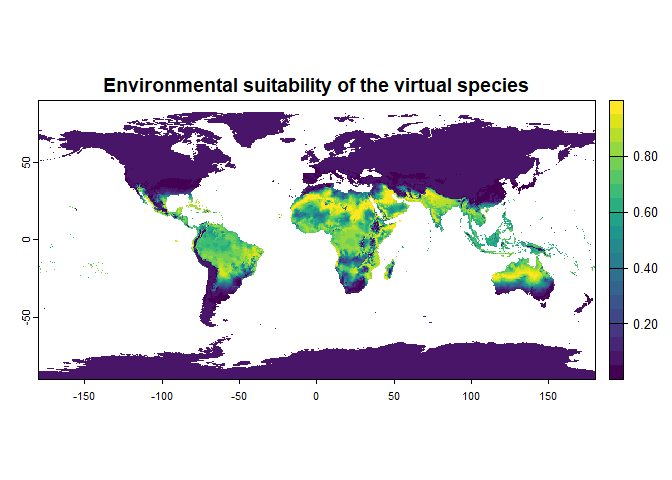Fig. 2.3 Environmental suitability of the generated virtual species

new.species
## Virtual species generated from 2 variables:
##  bio1, bio12
##
## - Approach used: Responses to each variable
## - Response functions:
##    .bio1  [min=-269; max=314] : dnorm   (mean=250; sd=50)
##    .bio12  [min=0; max=9916] : dnorm   (mean=4000; sd=2000)
## - Each response function was rescaled between 0 and 1
## - Environmental suitability formula = 3.1 * bio1^2 - 1.4 * sqrt(bio12) * bio1
## - Environmental suitability was rescaled between 0 and 1

Note that this is an example to show the possibilities of the function; I have no idea of the relevance of such a relationship!

### 2.2.4. Rescaling of the final environmental suitability

This final rescaling is performed because the combination of the different responses can lead to very different range of values. It is therefore necessary to allow environmental suitabilities to be comparable among virtual species, and should not be disabled unless you have very precise reasons to do it. The argument rescale controls this rescaling (TRUE by default).

## 2.3. How to create and use your own response functions

An important aspect of generateSpFromFun is that you can create and use your own response functions. In this section we will see how we can do that in practice.
We will take the example of a simple linear function: $f(x, a, b) = ax + b$

Our first step will be to create the function in R:

linear.function <- function(x, a, b)
{
a*x + b
}

That’s it! You created a function called linear.function in R.

Let’s try it now:

linear.function(x = 0.5, a = 2, b = 1)
##  2
linear.function(x = 3, a = 4, b = 1)
##  13
linear.function(x = -20, a = 2, b = 0)
##  -40

It seems to work properly. Now we will use linear.function to generate a virtual species distribution. We want a species responding linearly to the annual mean temperature, and with a gaussian to the annual precipitations:

my.responses <- formatFunctions(bio1 = c(fun = "linear.function", a = 1, b = 0),
bio12 = c(fun = "dnorm", mean = 1000, sd = 1000))

generateSpFromFun(raster.stack = worldclim[[c("bio1", "bio12")]],
parameters = my.responses, plot = TRUE)
## Generating virtual species environmental suitability...
##  - The response to each variable was rescaled between 0 and 1. To
##             disable, set argument rescale.each.response = FALSE
##  - The final environmental suitability was rescaled between 0 and 1.
##             To disable, set argument rescale = FALSE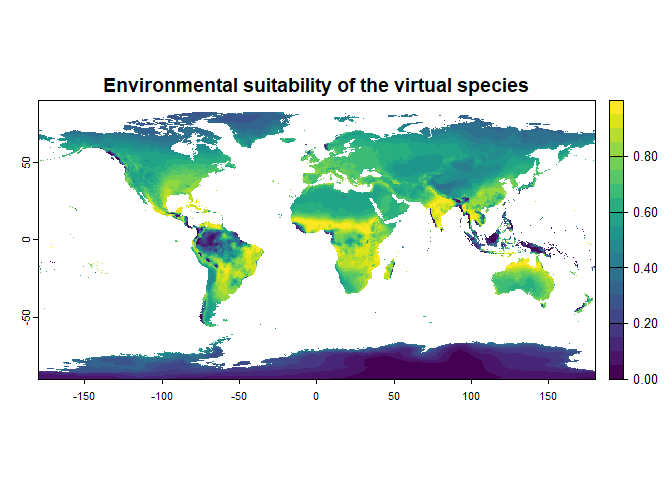Fig 2.3 Environmental suitability of the generated virtual species

## Virtual species generated from 2 variables:
##  bio1, bio12
##
## - Approach used: Responses to each variable
## - Response functions:
##    .bio1  [min=-269; max=314] : linear.function   (a=1; b=0)
##    .bio12  [min=0; max=9916] : dnorm   (mean=1000; sd=1000)
## - Each response function was rescaled between 0 and 1
## - Environmental suitability formula = bio1 * bio12
## - Environmental suitability was rescaled between 0 and 1

And it worked! Your turn now!

-----------------
Do not hesitate if you have a question, find a bug, or would like to add a feature in virtualspecies: mail me!